# Integral-transform method

Jump to: navigation, search

A method for solving linear differential equations for given boundary value or initial conditions, consisting in the transition from the given equation to an equation for an integral transform of the unknown function. The latter equation may turn out to be simpler. Suppose, e.g., that one has to find the solution to the equation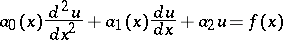(1)

on a finite or infinite interval with boundary conditions,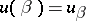. If the kernel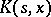of the integral transformation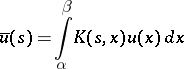satisfies the equation(2)

where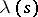is a function of, then after multiplication of (1) byand integration by parts over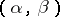one obtains the equation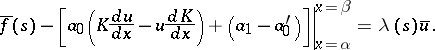Solving it forand using an inversion formula for the integral transformation, one finds. An analogous integral-transform method is used for partial differential equations.

Thus, the process for solving differential equations by this method consists of the following stages:

1) The choice of a suitable integral transformation.

2) Multiplication of the equation and boundary-initial conditions by the kernel of this integral transformation, and subsequent integration, over a suitable range, with respect to the independent variable.

3) In the integration of 2), the use of the boundary-initial conditions to compute the terms arising from the integration limits.

4) Solution of the auxiliary equation and search for the integral transform of the unknown function.

5) Determination of the unknown function by an inversion formula.